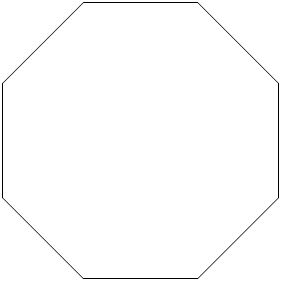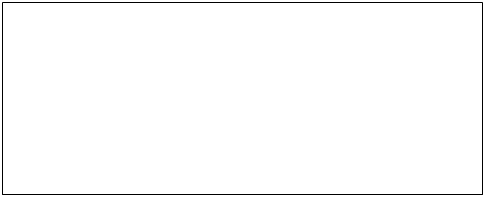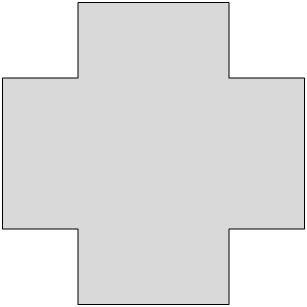# Shapes and Their Perimeter

## Objective

Find perimeter by measuring side lengths in whole number units.

## Common Core Standards

### Core Standards

?

• 3.MD.D.8 — Solve real world and mathematical problems involving perimeters of polygons, including finding the perimeter given the side lengths, finding an unknown side length, and exhibiting rectangles with the same perimeter and different areas or with the same area and different perimeters.

?

• 2.MD.A.1

• 3.OA.D.8

## Criteria for Success

?

1. Find the perimeter of a shape by tracing around the figure with string or pipe cleaners, then measuring the total length of the string or pipe cleaner (MP.5).
2. Find the perimeter of a shape by measuring the length of each side and adding their lengths together.
3. Write an equation to represent the perimeter of a shape (optionally including simplified equations, such as a multiplication sentence for a regular polygon) (MP.2).
4. Understand that perimeter is measured in length units (centimeters, inches, etc.) (MP.6).

#### Fishtank Plus

• Problem Set
• Student Handout Editor
• Vocabulary Package

?

### Problem 1

Use your ruler to find the perimeter, in inches, of the following shape.### Problem 2

Use your ruler to find the perimeter, in centimeters, of the following shape.### Problem 3

Use your ruler to find the perimeter, in inches, of the following rectangle.## Problem Set & Homework

#### Discussion of Problem Set

• Which shape has the smallest perimeter in #1? How do you know?
• What unit did you use to record the perimeters of the shapes in #1? Why?
• What do you notice about the perimeters of the shapes in #1(b) and 1(e)?
• I think that to find the perimeter of the shape in #2, I can find the perimeter of each triangle and add them together. Do you agree or disagree? Why? How does this problem challenge our understanding of perimeter?
• Whose shape has more sides in #3? Do more sides mean a greater perimeter? Why or why not?
• What multiplication number sentence can you use to find the perimeter of the square in #4?
• Explain to a partner how to use a ruler to find the perimeter of a shape.

?

Find the perimeter, in centimeters, of the shape below. Show or explain your work.#### References

EngageNY Mathematics Grade 3 Mathematics > Module 7 > Topic C > Lesson 12Exit Ticket

Grade 3 Mathematics > Module 7 > Topic C > Lesson 12 of the New York State Common Core Mathematics Curriculum from EngageNY and Great Minds. © 2015 Great Minds. Licensed by EngageNY of the New York State Education Department under the CC BY-NC-SA 3.0 US license. Accessed Dec. 2, 2016, 5:15 p.m..

Modified by The Match Foundation, Inc.

?